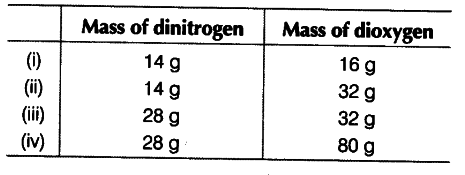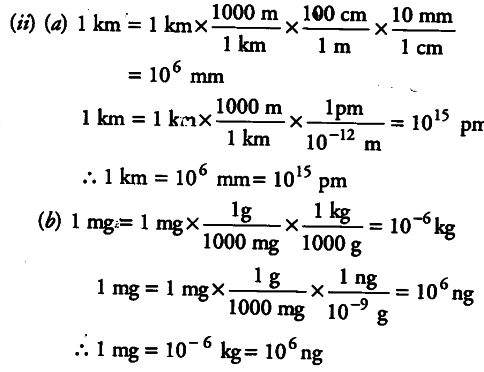# The following data are obtained when dinitrogen and dioxygen

The following data are obtained when dinitrogen and dioxygen react together to form different compounds.(i) Which law of chemical combination is obeyed by the above experimental data? Give statement. ’ 
(ii) Fill in the blanks in the following conversion.
(a) 1 km = … mm = … pm
(b) 1 mg = …kg = …ng
© 1 mL = … L = … {{dm}^{3}}

(i) On fixing the mass of dinitrogen as 28 g, the masses of dioxygen combined are 32, 64, 32 and 80 in the given four oxides. These are in the simple whole number ratio i.e., 2 : 4 : 2 : 5. Hence, the given data obey the law of multiple proportions.